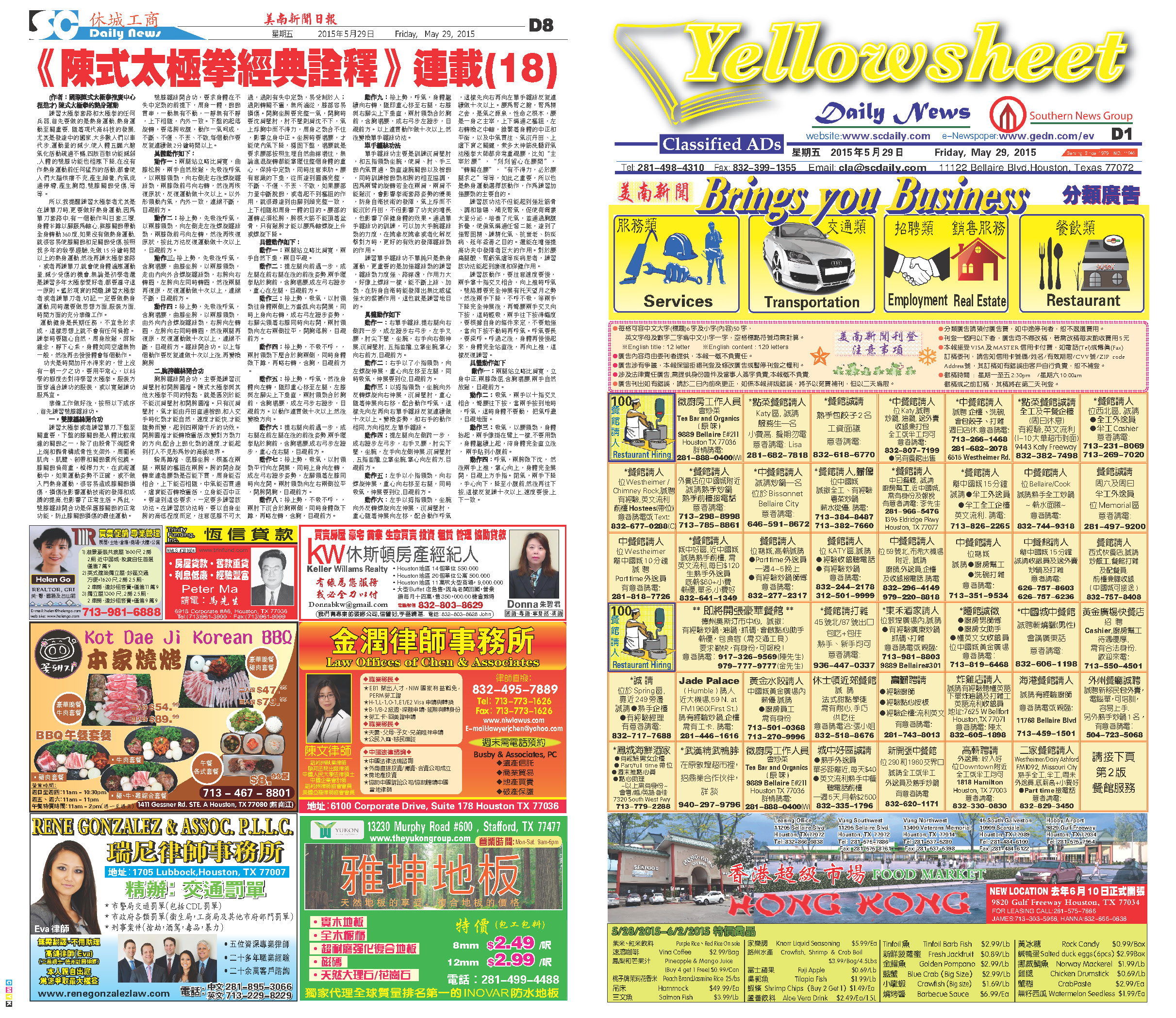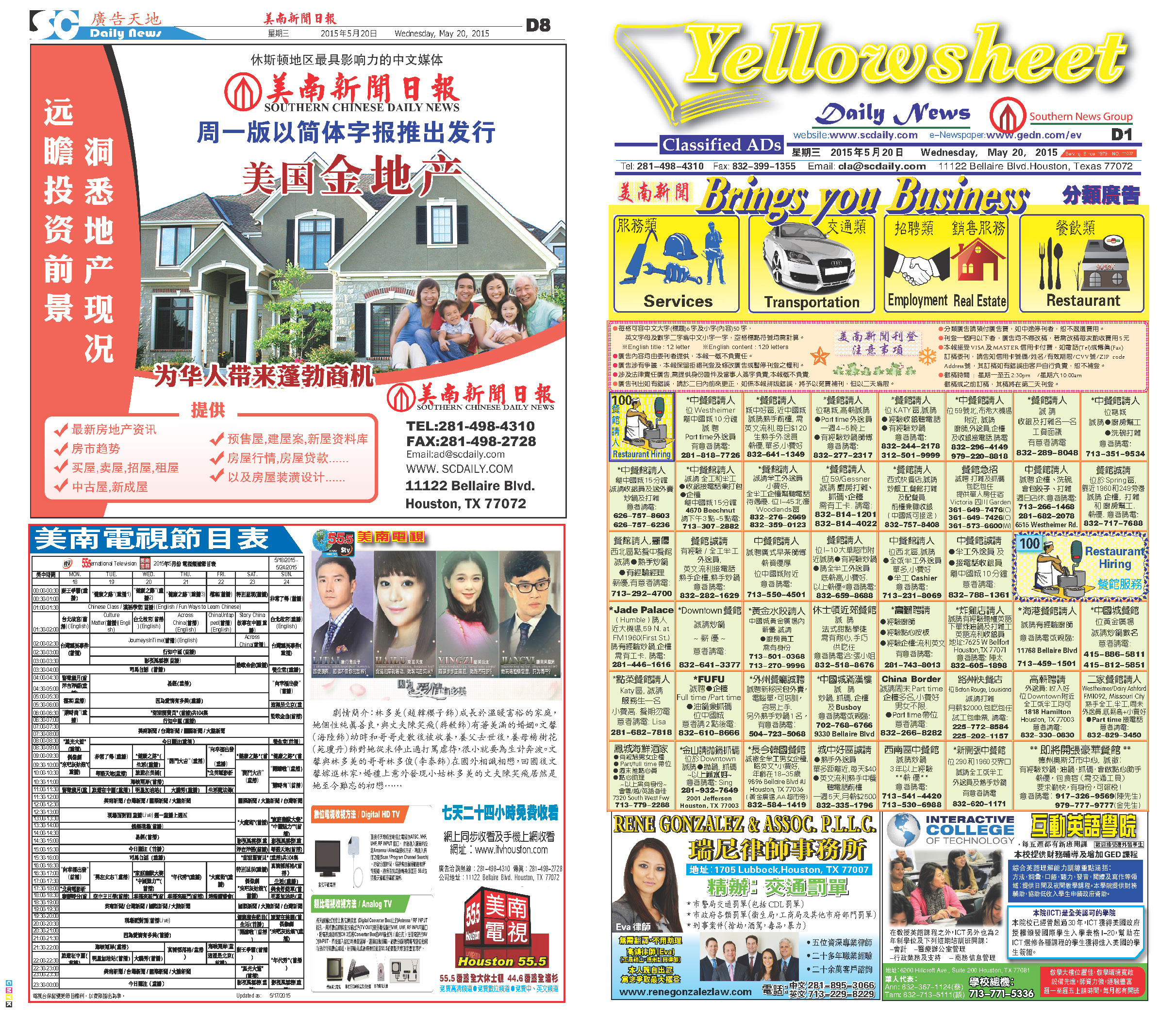150530 Epaper

 A Section B SectionC Section D Section150529 Epaper

 A Section B SectionC Section D Section150528 Epaper

 A Section B SectionC Section D Section150527 Epaper

 A Section B SectionC Section D Section150526 Epaper

 A Section B SectionC Section D Section150525 Epaper

 A Section B SectionC Section D Section150524 Epaper

 A Section B SectionC Section150523 Epaper

 A Section B SectionC Section D Section150521 Epaper

 A Section B SectionC Section D Section150522 Epaper

 A Section B SectionC Section D Section150520 Epaper

 A Section B SectionC Section D Section